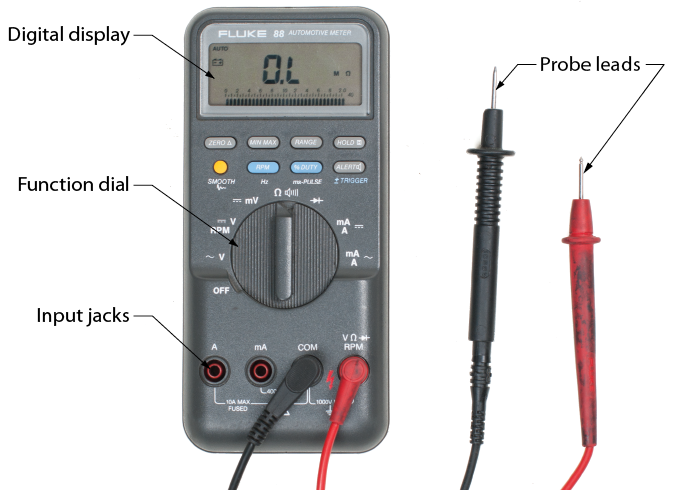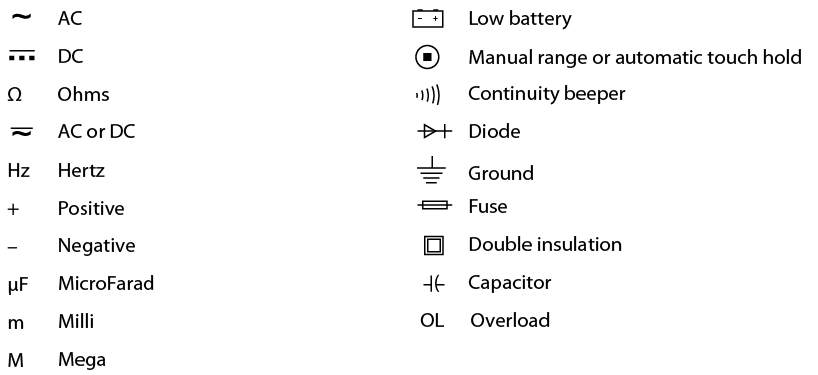# 12.3: Digital Multimeters

•• Camosun College
$$\newcommand{\vecs}{\overset { \rightharpoonup} {\mathbf{#1}} }$$ $$\newcommand{\vecd}{\overset{-\!-\!\rightharpoonup}{\vphantom{a}\smash {#1}}}$$$$\newcommand{\id}{\mathrm{id}}$$ $$\newcommand{\Span}{\mathrm{span}}$$ $$\newcommand{\kernel}{\mathrm{null}\,}$$ $$\newcommand{\range}{\mathrm{range}\,}$$ $$\newcommand{\RealPart}{\mathrm{Re}}$$ $$\newcommand{\ImaginaryPart}{\mathrm{Im}}$$ $$\newcommand{\Argument}{\mathrm{Arg}}$$ $$\newcommand{\norm}{\| #1 \|}$$ $$\newcommand{\inner}{\langle #1, #2 \rangle}$$ $$\newcommand{\Span}{\mathrm{span}}$$ $$\newcommand{\id}{\mathrm{id}}$$ $$\newcommand{\Span}{\mathrm{span}}$$ $$\newcommand{\kernel}{\mathrm{null}\,}$$ $$\newcommand{\range}{\mathrm{range}\,}$$ $$\newcommand{\RealPart}{\mathrm{Re}}$$ $$\newcommand{\ImaginaryPart}{\mathrm{Im}}$$ $$\newcommand{\Argument}{\mathrm{Arg}}$$ $$\newcommand{\norm}{\| #1 \|}$$ $$\newcommand{\inner}{\langle #1, #2 \rangle}$$ $$\newcommand{\Span}{\mathrm{span}}$$$$\newcommand{\AA}{\unicode[.8,0]{x212B}}$$

All digital multimeters combine the features of an ammeter, a voltmeter, and an ohmmeter. Since a DMM is an important tool, you will want to learn how to use one correctly. Figure $$\PageIndex{1}$$ shows a typical DMM, although different models may have a different number of digits in the display unit, and the input/output jacks may be in slightly different places.Figure $$\PageIndex{1}$$: Typical DMM (CC BY-NC-SA; BC Industry Training Authority)

The upper portion of the DMM houses the display unit. The middle portion of the DMM houses the function switch, and the bottom portion contains the jacks for test leads.

The function switch normally has positions that allow a technician to measure AC volts, DC volts, DC amps, and resistance. In addition, some DMMs have function switch positions that will allow a technician to measure AC amps, test diodes, and capacitors. Some DMMs require manual setting of ranges; others have an autoranging feature.

All DMMs may be used to measure voltage, current, and resistance. More advanced DMMs may measure frequency, relative power differences, or other important circuit parameters. Each measurement function has similarities and differences that you need to learn about.

Many meters will use symbols on the display, switch, and connections. Figure $$\PageIndex{2}$$ lists some of the common symbols you may see.Figure $$\PageIndex{2}$$: Common DMM symbols (CC BY-NC-SA; BC Industry Training Authority)